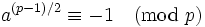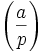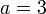# Proth's theorem

## Statement

### Existential version

Supposeis a Proth number, i.e., a number of the formwhereandis odd. Then,is a prime number (and hence a Proth prime) if and only if there existssuch that:Note that one direction of implication (prime implying the existence of) is true even for numbers that are not Proth numbers, so it is the other direction that is substantive.

### Particular element version

Supposeis a Proth number, i.e., a number of the formwhereandis odd. Pick an elementsuch that the Jacobi symbolis -1. Then,is a prime number (and hence a Proth prime) if and only if:## Related facts

• Pepin's primality test: This is a version for Fermat numbers. For Fermat numbers, we can choose.

## Proof

Fill this in later